CF809E Surprise me!

• 85通过
• 215提交
• 题目来源
• 评测方式 RemoteJudge
• 标签
• 难度 NOI/NOI+/CTSC
• 时空限制 8000ms / 256MB
• 提示：收藏到任务计划后，可在首页查看。

题意翻译

给定一棵 $n$ 个节点的树，每个点有一个权值 $a[i]$ ，保证 $a[i]$ 是一个 $1..n$ 的排列。 求 $$\frac{1}{n(n-1)}\sum_{i=1}^n\sum_{j=1}^n\varphi(a_i*a_j)·dist(i,j)$$ 其中， $\varphi(x)$ 是欧拉函数， $dist(i,j)$ 表示 $i,j$ 两个节点在树上的距离。

感谢@yybyyb 提供的翻译

题目描述

Tired of boring dates, Leha and Noora decided to play a game.

Leha found a tree with $n$ vertices numbered from $1$ to $n$ . We remind you that tree is an undirected graph without cycles. Each vertex $v$ of a tree has a number $a_{v}$ written on it. Quite by accident it turned out that all values written on vertices are distinct and are natural numbers between $1$ and $n$ .

The game goes in the following way. Noora chooses some vertex $u$ of a tree uniformly at random and passes a move to Leha. Leha, in his turn, chooses (also uniformly at random) some vertex $v$ from remaining vertices of a tree $(v≠u)$ . As you could guess there are $n(n-1)$ variants of choosing vertices by players. After that players calculate the value of a function $f(u,v)=φ(a_{u}·a_{v})$ $·$ $d(u,v)$ of the chosen vertices where $φ(x)$ is Euler's totient function and $d(x,y)$ is the shortest distance between vertices $x$ and $y$ in a tree.

Soon the game became boring for Noora, so Leha decided to defuse the situation and calculate expected value of function $f$ over all variants of choosing vertices $u$ and $v$ , hoping of at least somehow surprise the girl.

Leha asks for your help in calculating this expected value. Let this value be representable in the form of an irreducible fraction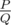. To further surprise Noora, he wants to name her the value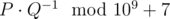.

Help Leha!

输入输出格式

输入格式：

The first line of input contains one integer number $n$ $(2<=n<=2·10^{5})$ — number of vertices in a tree.

The second line contains $n$ different numbers $a_{1},a_{2},...,a_{n}$ $(1<=a_{i}<=n)$ separated by spaces, denoting the values written on a tree vertices.

Each of the next $n-1$ lines contains two integer numbers $x$ and $y$ $(1<=x,y<=n)$ , describing the next edge of a tree. It is guaranteed that this set of edges describes a tree.

输出格式：

In a single line print a number equal to $P·Q^{-1}$ modulo $10^{9}+7$ .

输入输出样例

输入样例#1： 复制
3
1 2 3
1 2
2 3

输出样例#1： 复制
333333338

输入样例#2： 复制
5
5 4 3 1 2
3 5
1 2
4 3
2 5

输出样例#2： 复制
8


说明

Euler's totient function $φ(n)$ is the number of such $i$ that $1<=i<=n$ ,and $gcd(i,n)=1$ , where $gcd(x,y)$ is the greatest common divisor of numbers $x$ and $y$ .

There are $6$ variants of choosing vertices by Leha and Noora in the first testcase:

• $u=1$ , $v=2$ , $f(1,2)=φ(a_{1}·a_{2})·d(1,2)=φ(1·2)·1=φ(2)=1$
• $u=2$ , $v=1$ , $f(2,1)=f(1,2)=1$
• $u=1$ , $v=3$ , $f(1,3)=φ(a_{1}·a_{3})·d(1,3)=φ(1·3)·2=2φ(3)=4$
• $u=3$ , $v=1$ , $f(3,1)=f(1,3)=4$
• $u=2$ , $v=3$ , $f(2,3)=φ(a_{2}·a_{3})·d(2,3)=φ(2·3)·1=φ(6)=2$
• $u=3$ , $v=2$ , $f(3,2)=f(2,3)=2$

Expected value equals to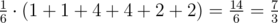. The value Leha wants to name Noora is $7·3^{-1}=7·333333336=333333338$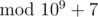.

In the second testcase expected value equals to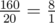, so Leha will have to surprise Hoora by number $8·1^{-1}=8$.

提示
标程仅供做题后或实在无思路时参考。
请自觉、自律地使用该功能并请对自己的学习负责。
如果发现恶意抄袭标程，将按照I类违反进行处理。